Question

# Suppose 5.00 mL of a 1.00 M stock solution is diluted to 25.00 mL. Afterwards, 5.00...

Suppose 5.00 mL of a 1.00 M stock solution is diluted to 25.00 mL. Afterwards, 5.00 mL of this solution is diluted to 25.00 mL. What is the concentration of the final solution?

Here we have to use the dilution formula in order to find the concentration.

Dilution formula: C1V1=C2V2 Where C= concentration and V= volume

Given, C1= 1.00M , V1= 5.00mL and V2= 25.00mL

On substituting; 1.00M X 5.00mL = C2 x 25.00mL

C2= 0.2M

Now 5.00mL of this 0.2M solution is diluted with 25.00 mL

So the concentration of final solution will be:

0.2M X 5.00mL = C2 X 25.00mL

C2= 0.04M

#### Earn Coins

Coins can be redeemed for fabulous gifts.

Similar Homework Help Questions
• ### ncetoi paper) 1. BSA stock solution A has a concentration of 3.50 mg/mL. 1.00 mL of...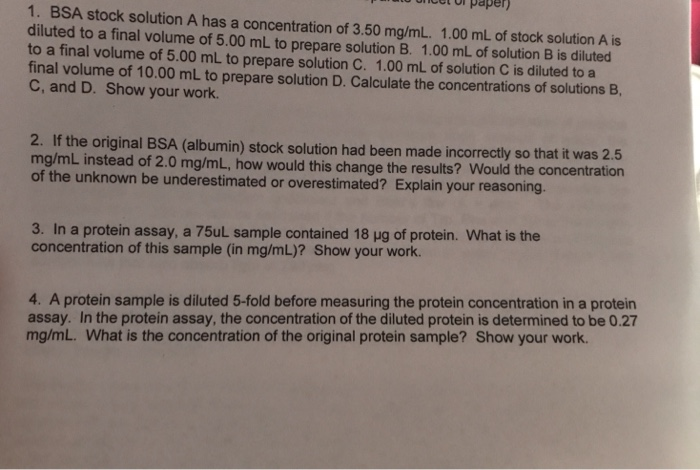ncetoi paper) 1. BSA stock solution A has a concentration of 3.50 mg/mL. 1.00 mL of stock solution A is diluted to a final volume of 5.00 mL to prepare solution B. 1.00 mL of solution B is diluted to a final volume of 5.00 mL to prepare solution C. 1.00 mL of solution C is diluted to a final volume of 10.00 mL to prepare solution D. Calculate the concentrations of solutions E, C, and D. Show your work....

• ### 50.0 mL of a stock solution of hydrochloric acid, HCl (aq), at 12.0 M is diluted...

50.0 mL of a stock solution of hydrochloric acid, HCl (aq), at 12.0 M is diluted by adding it to 150.0 mL of water, H2O. What is the concentration, in units of molarity, of the final, diluted solution?

• ### A student prepared a stock solution by dissolving 5.00 g of KOH in enough water to make 150. mL...

A student prepared a stock solution by dissolving 5.00 g of KOH in enough water to make 150. mL of solution. She then took 15.0 mL of the stock solution and diluted itwith enough water to make water to make 65.0 mL of a final solution. What is the concentration of KOH for the final solution?A) 0.137 MB) 7.30 MC)5.61 MD) 0.178 M

• ### a. A 10 ml calibration solution is made by mixing 5 mL of 0.200 M Fe(NO3)3,...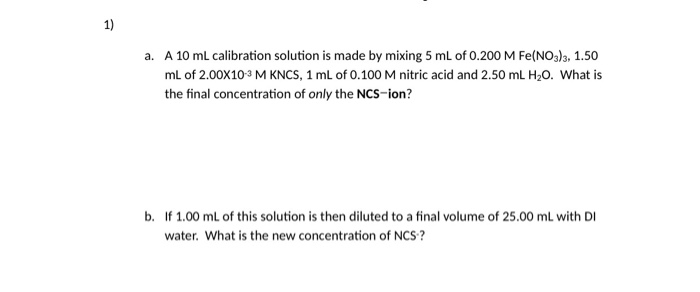a. A 10 ml calibration solution is made by mixing 5 mL of 0.200 M Fe(NO3)3, 1.50 mL of 2.00X10-3 M KNCS, 1 mL of 0.100 M nitric acid and 2.50 mL H20. What is the final concentration of only the NCS-ion? b. If 1.00 ml of this solution is then diluted to a final volume of 25.00 ml with DI water. What is the new concentration of NCS?

• ### if 25.00 ml of 0.275 M solution of AgNO3(aq) is mixed with 25.00 ml of 0.300...

if 25.00 ml of 0.275 M solution of AgNO3(aq) is mixed with 25.00 ml of 0.300 M CaCl2(aq), what will be the reaction? What is the final concentration of all of the ions after the two solutions are mixed?

• ### If 25.00 mL of 0.194 M NaOH is diluted to a volume of 100.0 mL, what...

If 25.00 mL of 0.194 M NaOH is diluted to a volume of 100.0 mL, what is the molarity of the resulting solution

• ### 1. Serial dilution Questions: a) (5 pts) Suppose you begin with stock solution of dye that has a concentration of 3...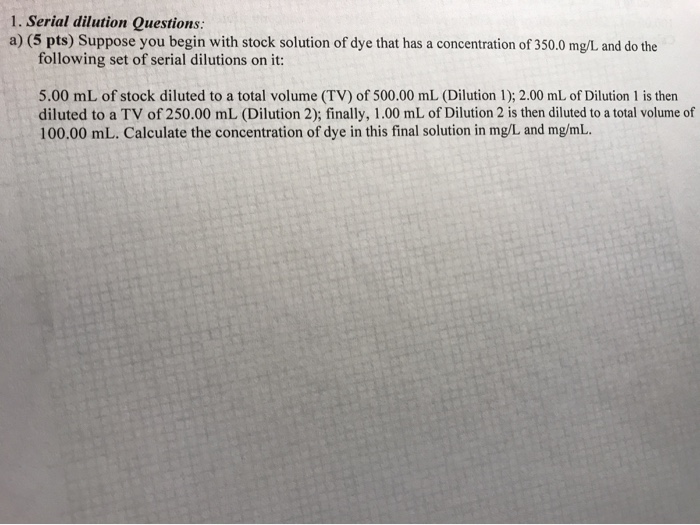1. Serial dilution Questions: a) (5 pts) Suppose you begin with stock solution of dye that has a concentration of 350.0 mg/L and do the following set of serial dilutions on it: 5.00 mL of stock diluted to a total volume (TV) of 500.00 mL (Dilution 1); 2.00 mL of Dilution 1 is then diluted to a TV of 250.00 mL (Dilution 2); finally, 1.00 mL of Dilution 2 is then diluted to a total volume of 100.00 mL. Calculate...

• ### Serial Dilution Questions 1, Serial dilution Questions: a) (5 pts) Suppose you begin with stock solution...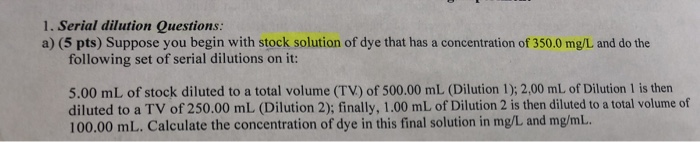Serial Dilution Questions 1, Serial dilution Questions: a) (5 pts) Suppose you begin with stock solution of dye that has a concentration of 350.0 mg/L and do the following set of serial dilutions on it: 5.00 mL of stock diluted to a total volume (TV) of 500.00 mL (Dilution 1); 2,00 mL of Dilution 1 is then diluted to a TV of 250.00 mL (Dilution 2); finally, 1.00 mL of Dilution 2 is then diluted to a total volume of...

• ### Suppose you have 100.00 mL of a solution of a dye and transfer 2.00 mL of...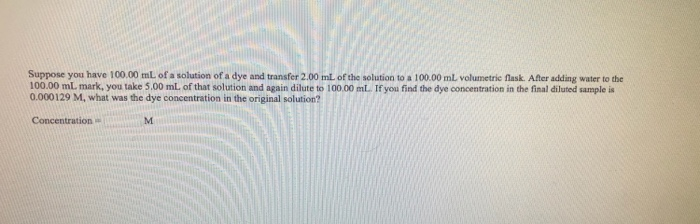Suppose you have 100.00 mL of a solution of a dye and transfer 2.00 mL of the solution to a 100.00 mL volumetric flask. After adding water to the 100.00 mL mark, you take 5.00 mL of that solution and again dilute to 100.00 mL. If you find the dye concentration in the final diluted sample is 0.000129 M, what was the dye concentration in the original solution? Concentration

• ### A 75.0 mL aliquot of a 1.50 M solution is diluted to a total volume of...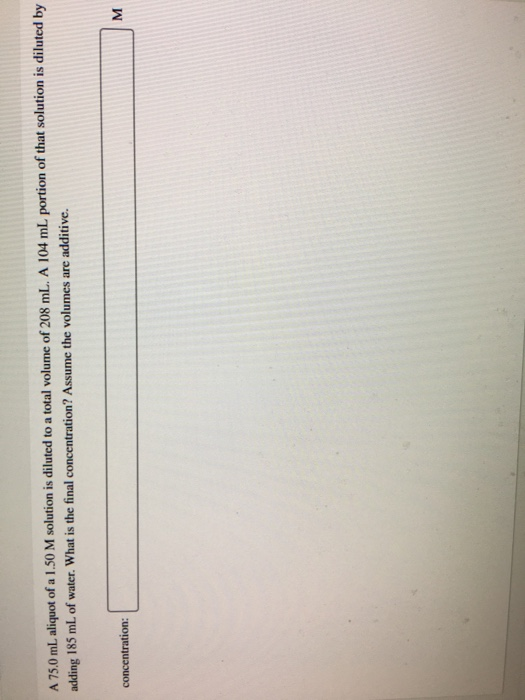A 75.0 mL aliquot of a 1.50 M solution is diluted to a total volume of 208 mL. A 104 mL portion of that solution is diluted by adding 185 mL of water. What is the final concentration? Assume the volumes are additive. concentration: M